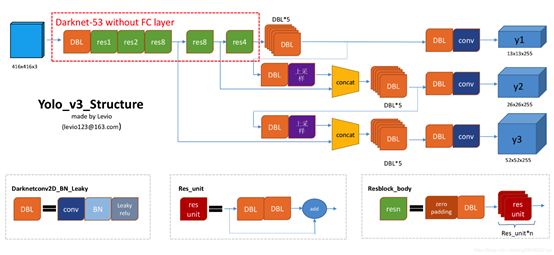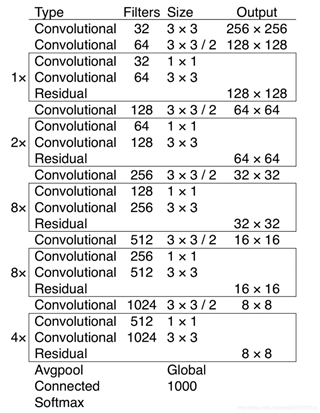# TensorFlow 2 实战之从零开始构建 YOLOv3 目标检测网络

1. 为什么选择学习 TensorFlow，以及如何安装 TensorFlow？
2. 为什么选择 YOLOv3 目标检测网络？
3. 深度学习前向推理的整个流程是什么样的？
4. 对 YOLOv3 网络构建部分进行详细的代码介绍。

## 1 Why TensorFlow ?

### 1.1 TensorFlow 特点

• 在开发语言方面，它支持 Python 开发、C++ 开发、JavaScript 开发、Swift 开发；

• 在模型开发方面，支持数据输入预处理、模型构建、模型训练、模型导出全流程；

• 在模型部署方面，支持在移动设备、嵌入式设备、服务端多种方式部署；

• 在硬件平台方面，支持在 CPU、GPU、TPU、RPI 等不同硬件上运行；

• 在开发工具方面，支持 TensorBoard 训练可视化、TensorFlow Hub 丰富的模型库

### 1.2 TensorFlow 安装

docker 可以有效的对环境进行隔离，容器中已经配置好了相关环境，可以免去很多手动安装操作，但对于 docker 操作不熟悉的同学来说，使用起来不太方便。

``````conda create -n tensorflow-gpu-2 python=3.7
conda activate tensorflow-gpu-2
pip install tensorflow-gpu
``````

## 2 Why YOLOv3?

YOLOv3 作为 one-stage 目标检测算法典型代表，在工业界应用非常广泛，尤其是对于实时性要求较高的场合，YOLOv3 及其各种变体基本上是第一选择。虽然现在已经有 YOLOv4 和 YOLOv5 更先进的算法，但也都是在 YOLOv3 的基础上改进而来，因此有必要先掌握 YOLOv3 再学习 YOLOv4 和 YOLOv5。

YOLOv3 原论文写得还是很“随意”的，很多技术细节没有讲，但这并不妨碍大家对他的肯定，关于对 YOLOv3 的解读，推荐两篇文章，分别是《深入浅出Yolo系列之 Yolov3 & Yolov4 & Yolov5 核心基础知识完整讲解》以及《你一定从未看过如此通俗易懂的 YOLO 系列（从 V1 到 V5 ）模型解读！》。

YOLOv3 整体结构如图所示，可以看到尺寸为 416 x 416 的输入图片进入 Darknet-53 网络后得到了 3 个分支，这些分支在经过一系列的卷积、上采样以及合并等操作后得到三个尺寸不一的 feature map，形状分别为 [13, 13, 255]、[26, 26, 255] 和 [52, 52, 255]，对这三个尺度的 feature map 经过一系列的后处理，即可得到输入图像的目标检测框。YOLOv3 主要有以下几个特点：

1. 采用 darknet53 作为特征提取网络；
2. 多尺度预测，输出三个分支，分别是 8 倍、16 倍、32 倍下采样，分别实现对小目标、中目标、大目标的检测；
3. 每个尺度使用三种宽高比的 anchor 进行预测，所以总共包含 9 个 anchor;
4. 网络的三个基本组件，分别是 DBL、res unit、rexn，具体细节参考上图;
5. 没有池化层和全连接层，通过改变卷积核的步长来调整张量的尺寸；

## 3 Show me code

### 3.1 总体流程

1. 定义模型输入
2. 定义模型输出
3. 加载权重文件
4. 准备输入数据
5. 模型前向推理
6. 输出后处理
7. 结果可视化

``````# 1、定义模型输入
input_size   = 416
input_layer  = tf.keras.layers.Input([input_size, input_size, 3])

# 2、定义模型输出
# 获得三种尺度的卷积输出
# 具体实现见 YOLOv3 函数说明
feature_maps = YOLOv3(input_layer)
bbox_tensors = []
# 依次遍历小、中、大尺寸的特征图
for i, fm in enumerate(feature_maps):
# 对每个分支的通道信息进行解码，得到预测框的大小、置信度和类别概率
# 具体操作见 decode 函数说明
bbox_tensor = decode(fm, i)
bbox_tensors.append(bbox_tensor)

# 3 加载权重文件
# 根据上边定义好的输入输出，实例化模型
model = tf.keras.Model(input_layer, bbox_tensors)
# 加载权重文件
# 输出模型信息
model.summary()

# 4、准备输入数据
image_path   = "./docs/kite.jpg"
original_image      = cv2.cvtColor(original_image, cv2.COLOR_BGR2RGB)
original_image_size = original_image.shape[:2]
image_data = utils.image_preporcess(np.copy(original_image), [input_size, input_size])
image_data = image_data[np.newaxis, ...].astype(np.float32)

# 5、模型前向推理
pred_bbox = model.predict(image_data)
pred_bbox = [tf.reshape(x, (-1, tf.shape(x)[-1])) for x in pred_bbox]
pred_bbox = tf.concat(pred_bbox, axis=0)

# 6、输出后处理，
bboxes = utils.postprocess_boxes(pred_bbox, original_image_size, input_size, 0.3)
bboxes = utils.nms(bboxes, 0.45, method='nms')

# 7、结果可视化
image = utils.draw_bbox(original_image, bboxes)
image = Image.fromarray(image)
image.save("result.jpg")
# image.show()
``````

### 3.2 网络结构

``````  def YOLOv3(input_layer):
# 输入层进入 Darknet-53 网络后，得到了三个分支
route_1, route_2, conv = backbone.darknet53(input_layer)
# 见上图中的橘黄色模块(DBL)，一共需要进行5次卷积操作
conv = common.convolutional(conv, (1, 1, 1024,  512))
conv = common.convolutional(conv, (3, 3,  512, 1024))
conv = common.convolutional(conv, (1, 1, 1024,  512))
conv = common.convolutional(conv, (3, 3,  512, 1024))
conv = common.convolutional(conv, (1, 1, 1024,  512))
conv_lobj_branch = common.convolutional(conv, (3, 3, 512, 1024))
# conv_lbbox 用于预测大尺寸物体，shape = [None, 13, 13, 255]
conv_lbbox = common.convolutional(conv_lobj_branch, (1, 1, 1024, 3*(NUM_CLASS + 5)),
activate=False, bn=False)
conv = common.convolutional(conv, (1, 1,  512,  256))
# 这里的 upsample 使用的是最近邻插值方法，这样的好处在于上采样过程不需要学习，从而减少了网络参数
conv = common.upsample(conv)
conv = tf.concat([conv, route_2], axis=-1)
conv = common.convolutional(conv, (1, 1, 768, 256))
conv = common.convolutional(conv, (3, 3, 256, 512))
conv = common.convolutional(conv, (1, 1, 512, 256))
conv = common.convolutional(conv, (3, 3, 256, 512))
conv = common.convolutional(conv, (1, 1, 512, 256))
conv_mobj_branch = common.convolutional(conv, (3, 3, 256, 512))
# conv_mbbox 用于预测中等尺寸物体，shape = [None, 26, 26, 255]
conv_mbbox = common.convolutional(conv_mobj_branch, (1, 1, 512, 3*(NUM_CLASS + 5)),
activate=False, bn=False)
conv = common.convolutional(conv, (1, 1, 256, 128))
conv = common.upsample(conv)
conv = tf.concat([conv, route_1], axis=-1)
conv = common.convolutional(conv, (1, 1, 384, 128))
conv = common.convolutional(conv, (3, 3, 128, 256))
conv = common.convolutional(conv, (1, 1, 256, 128))
conv = common.convolutional(conv, (3, 3, 128, 256))
conv = common.convolutional(conv, (1, 1, 256, 128))
conv_sobj_branch = common.convolutional(conv, (3, 3, 128, 256))
# conv_sbbox 用于预测小尺寸物体，shape = [None, 52, 52, 255]
conv_sbbox = common.convolutional(conv_sobj_branch, (1, 1, 256, 3*(NUM_CLASS +5)),
activate=False, bn=False)
return [conv_sbbox, conv_mbbox, conv_lbbox]
``````

### 3.3 darknet53

darknet53 网络结构如图所示，主要是由卷积层、残差模块组成。``````  def darknet53(input_data):

input_data = common.convolutional(input_data, (3, 3,  3,  32))
input_data = common.convolutional(input_data, (3, 3, 32,  64), downsample=True)

for i in range(1):
input_data = common.residual_block(input_data,  64,  32, 64)

input_data = common.convolutional(input_data, (3, 3,  64, 128), downsample=True)

for i in range(2):
input_data = common.residual_block(input_data, 128,  64, 128)

input_data = common.convolutional(input_data, (3, 3, 128, 256), downsample=True)

for i in range(8):
input_data = common.residual_block(input_data, 256, 128, 256)

route_1 = input_data
input_data = common.convolutional(input_data, (3, 3, 256, 512), downsample=True)

for i in range(8):
input_data = common.residual_block(input_data, 512, 256, 512)

route_2 = input_data
input_data = common.convolutional(input_data, (3, 3, 512, 1024), downsample=True)

for i in range(4):
input_data = common.residual_block(input_data, 1024, 512, 1024)

return route_1, route_2, input_data
``````

### 3.4 decode 处理

YOLOv3 网络的三个分支输出会被送入 decode 模块对 feature map 的通道信息进行解码，输出的是预测框在原图上的 [x, y, w, h, score, prob]。

``````  def decode(conv_output, i=0):
"""
return tensor of shape [batch_size, output_size, output_size, anchor_per_scale, 5 + num_classes]
contains (x, y, w, h, score, probability)
"""

conv_shape       = tf.shape(conv_output)
batch_size       = conv_shape
output_size      = conv_shape

# 对 tensor 进行 reshape
conv_output = tf.reshape(conv_output, (batch_size, output_size, output_size, 3, 5 + NUM_CLASS))

# 按顺序提取[x, y, w, h, c]
conv_raw_dxdy = conv_output[:, :, :, :, 0:2] # 中心位置的偏移量
conv_raw_dwdh = conv_output[:, :, :, :, 2:4] # 预测框长宽的偏移量
conv_raw_conf = conv_output[:, :, :, :, 4:5] # 预测框的置信度
conv_raw_prob = conv_output[:, :, :, :, 5: ] # 预测框的类别概率

# 好了，接下来是画网格。其中，output_size 等于 13、26 或者 52
y = tf.tile(tf.range(output_size, dtype=tf.int32)[:, tf.newaxis], [1, output_size])
x = tf.tile(tf.range(output_size, dtype=tf.int32)[tf.newaxis, :], [output_size, 1])
xy_grid = tf.concat([x[:, :, tf.newaxis], y[:, :, tf.newaxis]], axis=-1)
xy_grid = tf.tile(xy_grid[tf.newaxis, :, :, tf.newaxis, :], [batch_size, 1, 1, 3, 1])
xy_grid = tf.cast(xy_grid, tf.float32) # 计算网格左上角的位置，即cx cy的值

# 根据上图公式计算预测框的中心位置
# 这里的 i=0、1 或者 2， 以分别对应三种网格尺度
pred_xy = (tf.sigmoid(conv_raw_dxdy) + xy_grid) * STRIDES[i] # 计算预测框在原图尺寸上的x y
pred_wh = (tf.exp(conv_raw_dwdh) * ANCHORS[i]) * STRIDES[i] # 计算预测框在原图尺寸上的w h
pred_xywh = tf.concat([pred_xy, pred_wh], axis=-1) # 拼接起来
pred_conf = tf.sigmoid(conv_raw_conf) # 计算预测框里object的置信度
pred_prob = tf.sigmoid(conv_raw_prob) # 计算预测框里object的类别概率

return tf.concat([pred_xywh, pred_conf, pred_prob], axis=-1)
``````

## 4 Summary